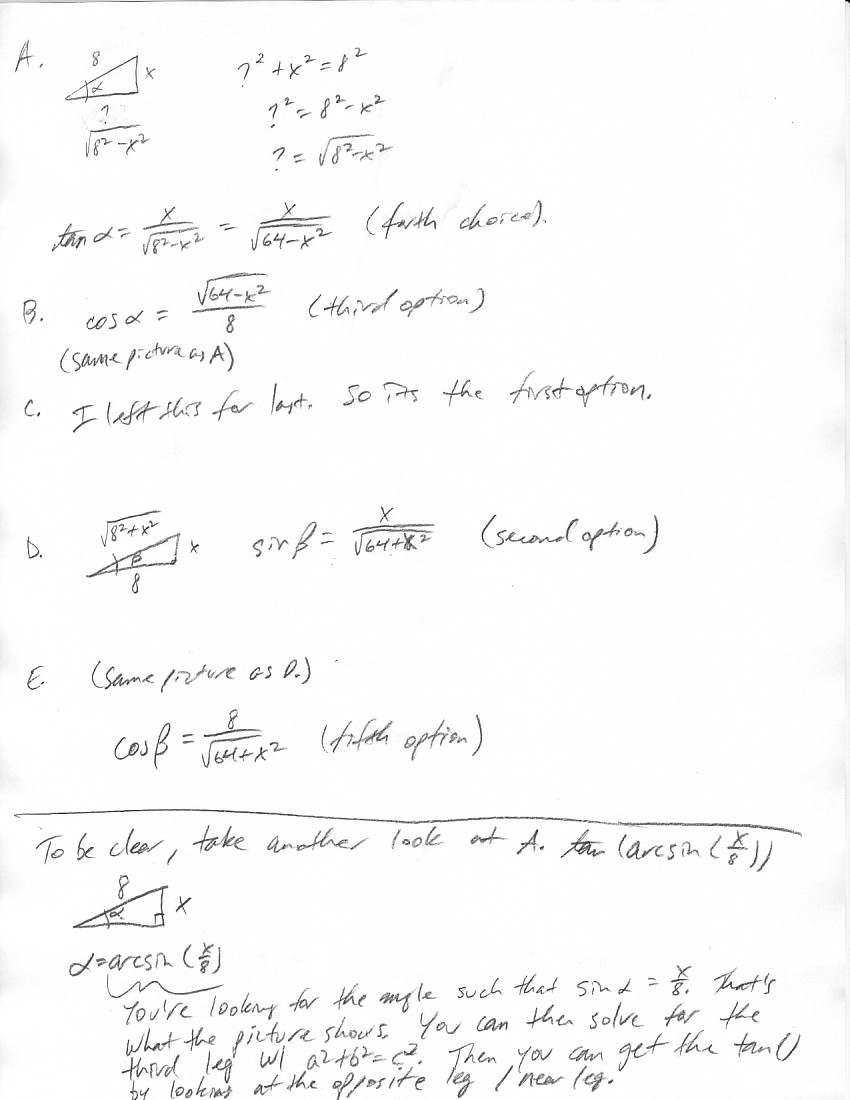# Match each of the trigonometric expressions below with the equivalent non-trigonometric function from the following list. Enter the appropriate lettermelodykap 2020-11-26 Answered
Match each of the trigonometric expressions below with the equivalent non-trigonometric function from the following list. Enter the appropriate letter(A,B, C, D or E)in each blank
A . $\mathrm{tan}\left(\mathrm{arcsin}\left(\frac{x}{8}\right)\right)$
B . $\mathrm{cos}\left(ar\mathrm{sin}\left(\frac{x}{8}\right)\right)$
C. $\left(\frac{1}{2}\right)\mathrm{sin}\left(2\mathrm{arcsin}\left(\frac{x}{8}\right)\right)$
D. $\mathrm{sin}\left(\mathrm{arctan}\left(\frac{x}{8}\right)\right)$
E. $\mathrm{cos}\left(\mathrm{arctan}\left(\frac{x}{8}\right)\right)$
You can still ask an expert for help

• Questions are typically answered in as fast as 30 minutes

Solve your problem for the price of one coffee

• Math expert for every subject
• Pay only if we can solve itCullen..